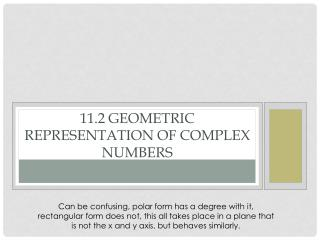DownloadDownload Presentation11.2 Geometric Representation of Complex Numbers

11.2 Geometric Representation of Complex Numbers

Download Presentation11.2 Geometric Representation of Complex Numbers

- - - - - - - - - - - - - - - - - - - - - - - - - - - E N D - - - - - - - - - - - - - - - - - - - - - - - - - - -
Presentation Transcript

1. 11.2 Geometric Representation of Complex Numbers Can be confusing, polar form has a degree with it, rectangular form does not, this all takes place in a plane that is not the x and y axis, but behaves similarly.

2. Complex numbers are numbers that involve i. • They are of the form a+bi. • We cannot graph these numbers on the Cartesian Plane because both axis on the Cartesian plane are representative of REAL numbers (not imaginary) • We do however have a method for graphing these complex numbers.

3. Jean Robert Argand • We can represent complex numbers geometrically thanks to Argand who made the argument that we can replace the y-axis with an imaginary axis . The following method will be how we plot complex numbers. Plot 6+5i. imaginary axis x axis This is called the complex plane or Argand Diagram.

4. This point that is representing a+bican be represented in rectangular coordinates (a,b) or in polar coordinates (r,ϴ). Lets see how we can work through the translation. In general we refer to the point by the name “z” Now that z=(a,b)=a+bi But in polar coordinates we know that a=rcosϴ and b=rsinϴ. So now replace a and b you get … rcosϴ + (rsinϴ)i Then you get rcos ϴ + r i sin ϴ…factor out r you get … r(cos ϴ+ i sin ϴ) We define (cos ϴ+i sin ϴ) as “cis” so z=rcis ϴ z = (a,b) In addition we can still find r by pythagoras, r or ||z||= r b ϴ a

5. Rewrite the following complex numbers in polar form. • 3-2i • -4+2i • -4i

6. Rewrite each complex number in rectangular form. • 8 cis 110 • 12 cis 250

7. Pg. 406 1-12

8. Product of 2 complex numbers in polar form • When we want to multiply two complex numbers we multiply the radii r and s, and we add the angles. i x

9. Express each product in polar form • (4 cis 25o)(6 cis 35o) = 24 cis (25o + 35o) =24 cis (60o) z1 z2 Point z2 a distance of 6 away from the origin and 35 degrees counterclockwise of the polar axis Point z1 a distance of 4 away from the origin and 25 degrees counterclockwise of the polar axis Point z1z2 a distance of 24 away from the origin and 60 degrees counterclockwise of the polar axis

10. Find z1z2 in rectangular form by multiplying z1 and z2. • Find z1, z2, and z1z2 in polar form. Show that z1z2 in polar form agrees with z1z2 in rectangular form. • Show z1, z2, and z1z2 in an Argand diagram.

11. HWK pg. 406 13-22